# Multiple ways to count texts in Excel

In this article, we will learn Multiple ways to count texts in Excel.

Scenario:

Microsoft Word allows you to count text, but what about Excel? If we need a count of our text values in data in Excel, we can get this done by using the COUNTIF function and then a combination of other functions and excel wildcards depending on our desired result. Let's understand how we can use the COUNTIF function for multiple purposes.

COUNTIF function to count different text in excel

If you want to learn how to count text in Excel, you need to use function COUNTIF with the criteria defined using wildcard ( * ) with quotes.

COUNTIF formula

 =COUNTIF(range, "*")

Here range is defined cell range where you want to count the text in Excel and wildcard * is criteria for all text occurrences in the defined range.

Wildcards

Wildcard characters in Excel

Question mark ( ? ) : This wildcard is used to search for any single character.

Asterisk ( * ): This wildcard is used to find any number of characters preceding or following any character.

Tilde ( ~ ): This wildcard is an escape character, used preceding the question mark ( ? ) or asterisk mark( * ).

Example :

All of these might be confusing to understand. Let's understand how to use the function using an example. Here Some interesting and very useful examples will be covered in this tutorial with the main focus on the COUNTIF function and different usages of this function in text counting. Limitations of COUNTIF function have been covered in this tutorial with an additional explanation of other functions such as SUMPRODUCT/ISNUMBER/FIND functions combination. After this tutorial, you will be able to count text cells in excel, count specific text cells, case sensitive text cells and text cells with multiple criteria defined – which is a very good base for further creative Excel problem-solving.

Here we have the ID records and we need to find the IDs which ends with A category.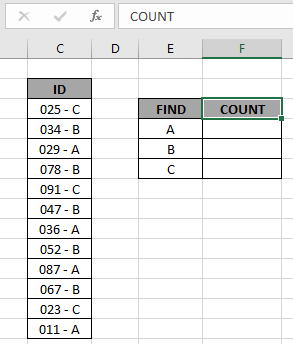Here to find the A category IDs, will be using the * (asterisk) wildcard in the formula. * (asterisk) wildcard finds any number of characters within lookup value.

Use the formula:

 = COUNTIF ( C3:C14, "*A" )

OR

 = COUNTIF ( C3:C14, "*" & E4 )

Explanation:

• COUNTIF function count the cells given criteria
• Criteria is given in using * (asterisk) wildcard to look for value which has any number of characters.
• & operator concatenate the two values where it lays
• "*" & E4 : criteria, formula checks each cell and returns only the cells which satisfies the criteria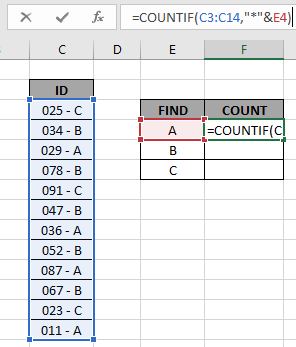Here the range is given as array reference and pattern is given as cell reference. Press Enter to get the count.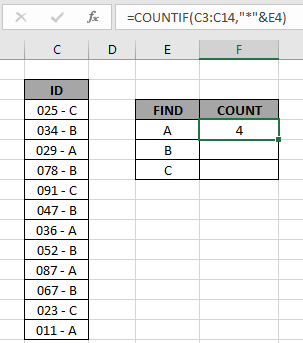As you can see the total values which ends with value A comes out to be 4.

Now copy the formula in other cells using the drag down option or using the shortcut key Ctrl + D as shown below.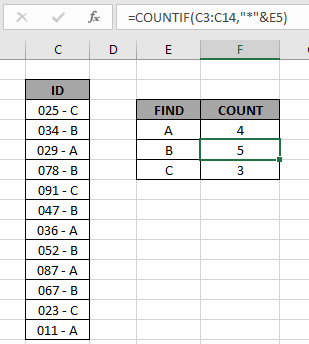As you can see all the different category counts of IDs are there.

COUNT cells if any one of the three values occur

There are different shades of colours in range ( A2:A13 ). We need to find the colours which have either Blue, red or violet.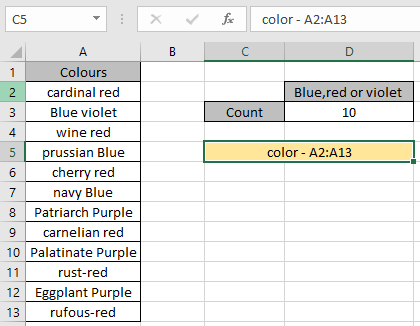Use the formula:

 = SUM ( COUNTIF ( color , { "*Blue*" ; "*red*" ; "*violet*" } ) )

Color : named range for the array A2:A13

* :  Asterisk ( * ) wildcard used to extract value wherever value is found.

The formula drops down to

= SUM ( { 3 ; 6 ; 1 } )

3 Blue, 6 red and 1 violet color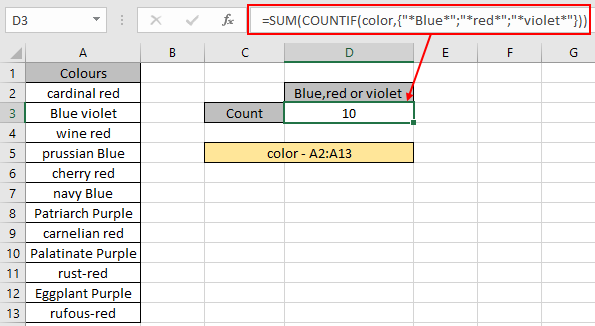There are 10 colors which have either Blue, red or violet.

As you can see the COUNTIF formula returns the count of cells having either of A, B or C value.

COUNTIFS to match multiple criteria

Here we have the ID records and we need to find the COUNT of ID values where respective numbers lays between the given range.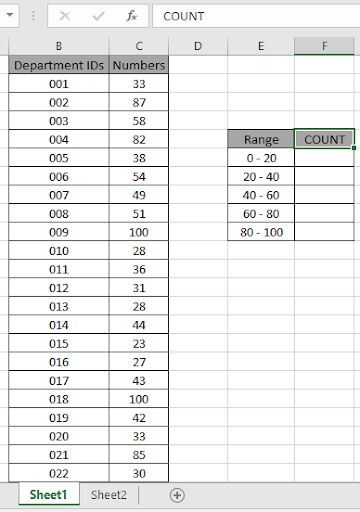Here to find the values in range. Some range references given using named range.

Numbers array given as Value named range for the array C3:C24.

Use the formula:

 = COUNTIFS ( Value , ">=0" , Value , "<20" )

Explanation:

• COUNTIFS function counts the cells that match the numbers in the Value array which are greater than equals to 0 (0 included).
• COUNTIFS function counts the cells that match the numbers in the Value array which are less than 20 (20 excluded).
• The formula counts the cell which satisfies both conditions which means value which lay between 0 to 20 will be counted.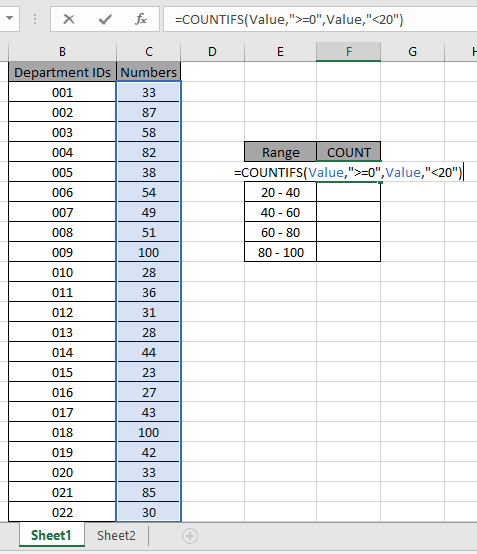The formula looks like as shown in the above snapshot. The Value array is given as a named range.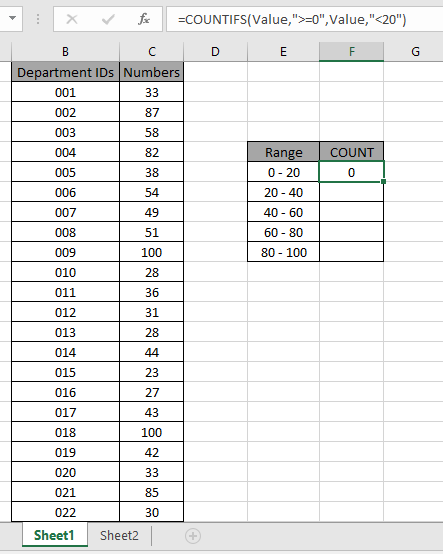As you can see the total values which occur between 0 - 20 comes out to be 0 i.e. there are no such values between 0 - 20.

Now copy the formula in other cells using the drag down option or using the shortcut key Ctrl + D as shown below. And changing the range parameter to 20 - 40.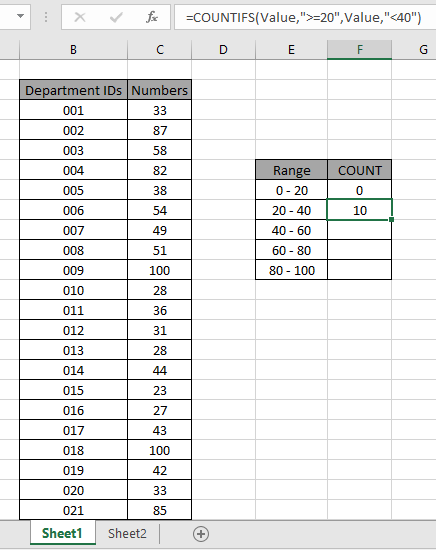As you can see values which lay between 20 - 40 are 10.

Now calculate all the other counts with given range values as explained above.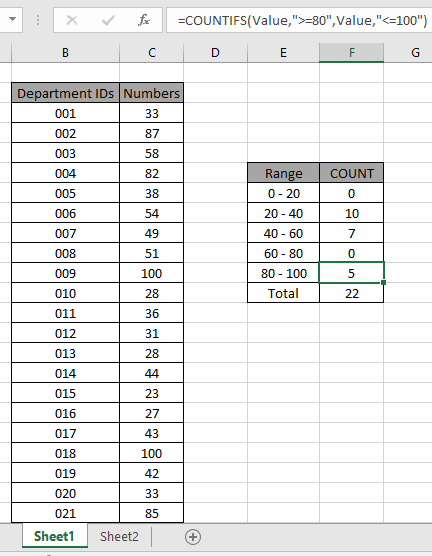We obtained all the counts between given range as it also proves the formula works fine.

Another Example:

We have this data for our next COUNTIFS example.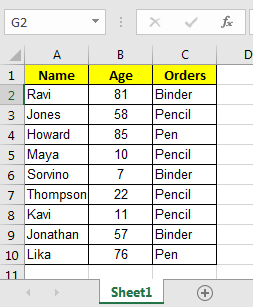And, we have these two queries to answer.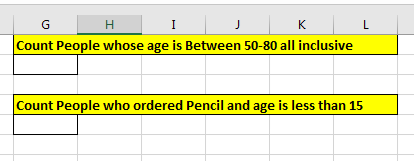So for the first query we have two conditions from one single column, Age.

To answer the query, Count People whose age is Between 50-80 all inclusive, write this COUNTIFS formula.

 =COUNTIFS(B2:B10,">=50",B2:B10,"<=80")

Note that, we have the same criteria range B2:B10  for both conditions. Excel has no objection to the use of the same criteria ranges. With the picked data output will be 3

How it Works?

Simple, the k function will first look for all values in range B2:B10 that will be {81,58,85,57,76}. Then in the {81,58,85,57,76} list it will look at all values that are less than or equal to 80 and that will be {58,57,76}. And that is 3.

Now in the second query in which I need to Count People who ordered Pencil and age is less than 15. We have to count on multiple criteria and different columns.

So  two answers to this query write this COUNTIFS formula.

 =COUNTIFS(C2:C10,"Pencil",B2:B10,"<15")

Note that we used two different columns for our condition.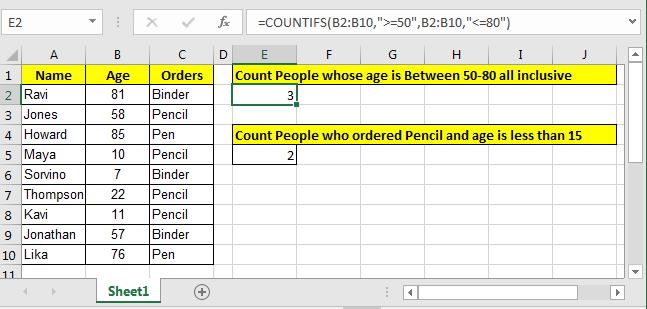Well this is the  classic way of counting in excel for two or more criteria. But there is an alternative way to count if two criteria match.

Count Users If Dealer Code Matches Or Year Using SUMPRODUCT

Here we have a data set of salespeople. The data contains many columns. What we need to do is to count the number of users who have code "INKA" or year is "2016". Make sure that if someone has both (code as "inka" and year 2016) should be counted as 1.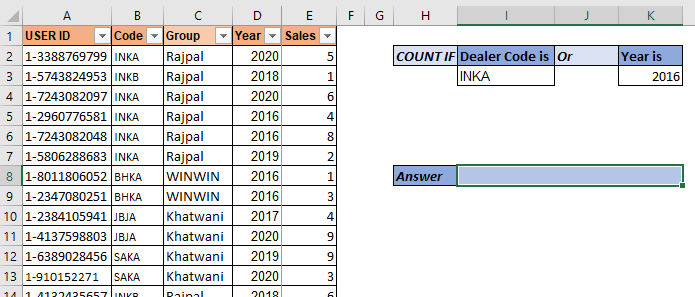So, hear we have two criteria. We use the above mentioned SUMPRODUCT formula:

 =SUMPRODUCT(--(((Code=I3)+(Year=K3))>0))

Here, code and year are named ranges.

This returns 7.

In the data we have  5 records of INKA code and 4 records of year 2016. But 2 records have both "INKA" and 2016 as code and year respectively. And these records are counted as 1. And this is how we get 7.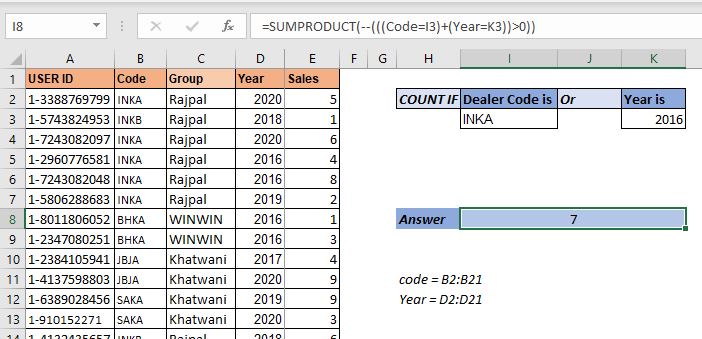So let's have a look at how the formula is solved step by step, then I will discuss how it works.

• SUMPRODUCT(--(((Code=I3)+(Year=K3))>0))
• SUMPRODUCT(--(({TRUE;FALSE;TRUE;TRUE;TRUE;TRUE;...}+{FALSE;FALSE;FALSE;TRUE;TRUE;...})>0))
• SUMPRODUCT(--(({1;0;1;2;2;1;1;1;0;0;0;0;0;0;0;0;0;0;0;0})>0))
• SUMPRODUCT(--({TRUE;FALSE;TRUE;TRUE;TRUE;TRUE;TRUE;...})
• SUMPRODUCT({1;0;1;1;1;1;1;1;0;0;0;0;0;0;0;0;0;0;0;0})
###### 7

In the first step, the value of I3 ("INKA") is compared with each cell in the code range. This returns an array of TRUE and FALSE. TRUE for each match.  To save space I have not shown all TRUE-FALSE. Similarly the value of K3 (2016) is matched with each cell in the year range.

In the next step, we add these two arrays that result into a new array of numeric values. As you may know that TRUE is treated as 1 and FALSE as 0 in Excel. So when TRUE and TRUE are added we get 2 and rest you can understand.

In the next step, we check which value is greater than 0 in the array. This again converts the array into a true false array. For each 0 value we get, False and rest are converted as true. Now the number of TRUE values in the array is our answer. But how do we count them? Here's how.

The double negative (--) signs are used to convert boolean values into 1s and 0s. So each TRUE value in the array is converted into 1 and FALSE into 0.

In the final step the sumproduct sums up this array and we get our answer as 7.

Adding More Or Criterias To Count Using SUMPRODUCT

So if you need to add more or criterias to count, you can just add criteria using + sign to the function.

For example, if you want to add another criteria to the above formula so that it adds the number of employees who have sold more than 5 products. The SUMPRODUCT formula will simply look like this:

 =SUMPRODUCT(--(((Code=I3)+(Year=K3)+(Sales>5))>0))

Simple! isn't it?

But let's say you want to have two criterias from Code range. Let's say you want to count "INKB". Then how do you do this? One method is using the above technique but that would be repetitive. Let's say I want to add 10 more criterias from the same range. In such cases this technique is not that smart for counting with SUMPRODUCT.

Let's say we have data arranged like this.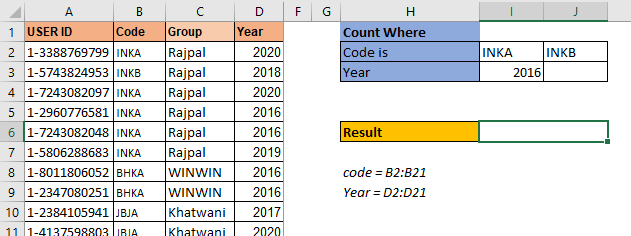The criteria codes are in one row I2:J2. The arrangement of data is important here. The SUMPRODUCT formula for 3 OR criteria count setting will be:

 =SUMPRODUCT(--(((Code=I2:J2)+(Year=I3:J3))>0))

This is the SUMPRODUCT formula to count with multiple criteria when multiple criteria from one range is written in row.

This returns the correct answer which is 10.

If you type any year in J3, the formula will add that count too.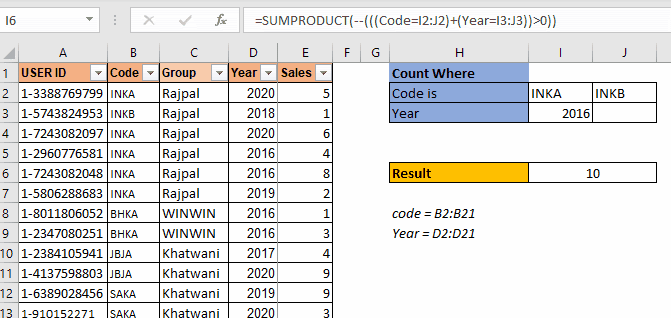This is used when the criterias are in one row. Will it work when the criterias in one column for the same range? No. It won't.

In this example we have multiple codes to count but these type codes are written in one column. When we use the above SUMPRODUCT formula, we get ans #N/A error. We will not get into how this error comes as this makes this article too long. Let's see how we can make this work.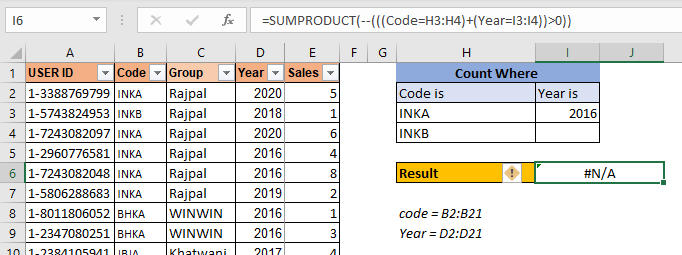To make this formula work, you need to wrap the code criteria in TRANSPOSE function. This will get the formula working.

 =SUMPRODUCT(--(((Code=TRANSPOSE(H3:H4))+(Year=TRANSPOSE(I3:I4)))>0))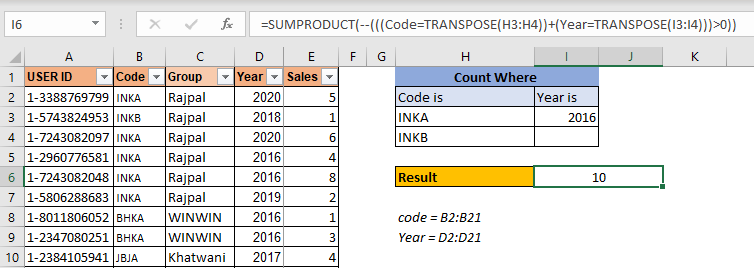This is the formula for counting with multiple or conditions in the same range when criteria is listed in a column.

Here are all the observational notes using the formula in Excel
Notes :

1. The formula only works with numbers and text both.
2. The formula ignores text value while comparing numbers and ignores numbers when it matches text values.
3. Operators like equals to ( = ), less than equal to ( <= ), greater than ( > ) or not equals to ( <> ) can be performed within a function applied with number only.

Hope this article about Multiple ways to count texts in Excel is explanatory. Find more articles on calculating values and related Excel formulas here. If you liked our blogs, share it with your friends on Facebook. And also you can follow us on Twitter and Facebook. We would love to hear from you, do let us know how we can improve, complement or innovate our work and make it better for you. Write to us at info@exceltip.com.

Related Articles :

How to use the SUMPRODUCT function in Excel: Returns the SUM after multiplication of values in multiple arrays in excel. This function can be used to do multiple tasks. This is one of the most versatile functions.

COUNTIFS with Dynamic Criteria Range : To count with dynamic criteria range we simply use the INDIRECT function. This function can

COUNTIFS With OR For Multiple Criteria : Count cells having multiple criteria match using the OR function. To put an OR logic in COUNTIFS function you will not need to use the OR function.

Using the IF with AND / OR Functions in Microsoft Excel : These logical functions are used to carry out multiple criteria calculations. With IF the OR and AND functions are used to include or exclude matches.

How to use OR function in Microsoft Excel : The function is used to include all the TRUE values in multiple criterias.

How to Count Cells That Contain This Or That in Excel in Excel :To cells that contain this or that, we can use the SUMPRODUCT function. Here's how you do those calculations.

Popular Articles :